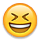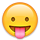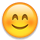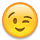# Conversion between W/Hr and A/Hr for running appliance off battery.

I'm having a little bit of difficultly looking through tons of threads about running things off battery. Is there an easy way to figure out how much an appliance uses, for easy of discussion, let's use 120v, and uses 100w. Now, I don't have the KWh rating for my exact design, but similar unit online says 200KWh/yr, meaning 0.55KW/day and 0.023KW/hour...right?

Ok, so if the 0.023kw an hour is for 120, how would I know what battery would maintain it for 10 hours? Ohm's law is stating that 120v, 2.2w/hr is 0.018A??
I can not math well, so I have a feeling I'm missing something here, because a I would only need 1AH battery to run this for 10hr.
Well, plus there are losses in 12vdc to 120vac inverter. Which is, how much?

Why can't this be simple?

ps: let's ignore solar input right now, and focus on how to convert a known appliance tag rating, to a battery running it all day, while being charged.

or did I just open a can of worms that will make my brain explode?

• Re: Conversion between W/Hr and A/Hr for running appliance off battery.

Welcome to the forum.

"Simple" is a relative term. Compared to nuclear physics it's pretty simple.First problem: the numbers supplied by the manufacturer are a lie. Kw hours per year are based on lab testing, which is not the real world. The Amps shown on the equipment tag are a maximum, not a running or peak or average. About the only thing that will be right is the Voltage. Even then they may give a range. The refrigerator doesn't run all the time either.

Solution: plug it in to a Kill-A-Watt meter and see what it really uses. The longer it's plugged in, the more accurate the data. We're talking typical average use numbers here; not some lab ideal condition results.

Second problem: inverters consume power themselves and are somewhat inefficient at converting the DC to AC.

Solution: once you've got a real number for the 'frige, look at the numbers for the inverter. They will have an efficiency rating (typically 90%) which needs to be factored in to the load consumption. Then you'll have the Watt hours for the load in DC. Then you add in the inverter's own consumption, and divide by the system Voltage to get the approximate Amp hours used.

Third problem: note I said "approximate". Batteries do operate at a fixed Voltage but rather over a range. As that Voltage goes down the current goes up to provide the equivalent Watts. Greater current draw = less actual battery capacity available.

Solution: err on the side of caution. Assume the load will draw more, the inverter will be less efficient than claimed, limit the Voltage to battery nominal, expect the batteries to be on the low side of their rated capacity (they will decline in time no matter what).

Trying to do it with the "actual" math will give you an unending headache. That's why there are shortcuts that will make the calculations easier and get you a functioning system better than 90% of the time.
Re: Conversion between W/Hr and A/Hr for running appliance off battery.
Riff42 wrote: »
I'm having a little bit of difficultly looking through tons of threads about running things off battery. Is there an easy way to figure out how much an appliance uses, for easy of discussion, let's use 120v, and uses 100w. Now, I don't have the KWh rating for my exact design, but similar unit online says 200KWh/yr, meaning 0.55KW/day and 0.023KW/hour...right?
• 200 kWH per year * 1/365 days per year * 1/24 hours per day = 0.023 kWatt = 23 Watt average load
Ok, so if the 0.023kw an hour is for 120, how would I know what battery would maintain it for 10 hours? Ohm's law is stating that 120v, 2.2w/hr is 0.018A??

Missed a decimal point there--it is an average of 23 watt load.
• 23 watts * 10 hours = 230 Watt*Hours of 120 VAC load

Now, for some rules of thumb. We recommend discharging a battery between ~25% to 50% per load cycle (you want 10 hours--Normally, for off grid systems we recommend 1-3 days of "no sun" storage--With 2 days as a "healthy" number for a balanced storage system)... But, anyway, say you have utility power and want to have a battery back up system for 10 hours per day (summer afternoon brownouts in the islands, etc.).

So, nominally, with a 25% discharge per 10 hour period and 85% AC inverter efficiency on a 12 volt battery bank:
• 23 watts * 10 hours * 1/0.85 inverter efficiency * 1/0.25 battery capacity * 1/12 volt battery bank = 90 AH @ 12 volt battery bank "nominal"

Note that many loads (such as a refrigerator) cycle... So a refrigerator running an average of 23 watts may actually run 20% of the time:
• 23 watts average * / 0.20 = 115 watts estimated "average run watts"

So the battery+AC Inverter+DC wiring need to be able to support the average run power of 115 watts plus something like 5x that amount for starting surge (most "good quality" inverters will support something like 2x their rated output for surge).
I can not math well, so I have a feeling I'm missing something here, because a I would only need 1AH battery to run this for 10hr.
Well, plus there are losses in 12vdc to 120vac inverter. Which is, how much?

Besides missing the decimal point (2.3 watts vs 23 watts)... You may be confusing the 120 VAC side vs the 12 VDC side of the calculations...
• Watts = Power = Voltage * Current

So, "Watts" is a "complete" unit--It fully describes the power used.

For Amps, which is used a lot when talking about DC power/battery banks/etc., is used a lot for "power" calculations but we need to need to know the "Volts" too for the full math. For example:
• 23 watts * 1/120 VAC = 0.19 amps @ 120 VAC
• 23 watts * 1/12 VDC = 1.9 amps @ 12 VDC

So, notice that the current at 12 VDC is 10x that of the "120 VAC side" of the system...

The summary is, missed the decimal point--10x factor missing. And (I think) confusing 23 watts at 120 VAC vs 12 VDC is another factor of 10...
• Your "1 AH battery" becomes 10*10*1AH = 100 AH @ 12 vdc...
Why can't this be simple?

It is "simple" math--But many folks (including me) hate word problems. I try to write my math equations like English sentences (notice my English ain't so good either--And I failed finger painting--what do I have left). So you can "see" all of the factors that go into the overall calculations.
ps: let's ignore solar input right now, and focus on how to convert a known appliance tag rating, to a battery running it all day, while being charged.

or did I just open a can of worms that will make my brain explode?

No--Just don't be afraid of the math... It is just a series of factors multiplied out. If you want to do the same thing with a 24 volt battery bank, just change the battery voltage factor. If you want to discharge the battery to 50% every 10 hour cycle, just change out to 0.50 battery cycle factor (and magically, your battery is 1/2 the AH rating to 45 Amp*Hours).

One other confusing point for most people... Watts is a rate (power) like miles per hour. Watt*Hours is an "amount" of "energy" like Miles driven.

So, the 23 watts (power) * 10 hours (time) = 230 Watt*Hours (amount)

People want to type 23 Watts per Hour --- But that is "wrong" as a Watt is already a unit of energy per unit time (Joules per Second). If you go back to your schooling, keep track of your units--They should all cancel out at the end (and remember that Watts=Volts*Amps):
• 23 Volts*Amps (watts) * 1/12 Volts = 1.9 Amps (dividing by volts leaves you with Amps as the output unit)

Note that Amp*Hour can be translated to Watt*Hour by multiplying the voltage (note that "k" is a factor of 1,000x):
• 90 AH battery bank * 12 Volt Battery = 1,080 VAH = 1,080 Watt*Hour = 1.08 kWH of storage

For measuring AC loads--It is hard to beat a Kill-a-Watt type meter. You can get "whole house" kWH meters. And you can get DC Amp*Hour/Watt*Hour meters too. And an AC/DC Current Clamp DMM (digital multi meter) is real handy too.

Get a Kill-a-Watt type meter and measure your AC appliances (plug in K-a-W meter for 1-7 days to each appliance) and "do the math"--You will quickly find which appliances are "killers" on your power bill and/or for your off grid system.

Hope that helps.

-Bill
Near San Francisco California: 3.5kWatt Grid Tied Solar power system+small backup genset
• Re: Conversion between W/Hr and A/Hr for running appliance off battery.

Well sweet jesus BB, thank you so much! It does clear up A LOT of information I have been missing. I do have a Kill-a-watt meter plugged into it right now actually to get a duty load rating, and then I can go from there I believe!!

Now to figure out if I can buy a motor, fan, panel and switch and wire up a solar power gable roof exhaust fan, instead of buying one for nearly twice as muchI'll come back to this thread soon with numbers and see if I did the math right for panel/battery needs.
Re: Conversion between W/Hr and A/Hr for running appliance off battery.

You are very welcome Riff... Feel free to come back any time and post your questions/assumptions and we will go from there.

You can post to this "your" thread--Or create a new one.

In general, we like to keep a single thread for questions/answer/discussions about a single system--Makes it easier to back a few posts to catch up on the discussion.

-Bill
Near San Francisco California: 3.5kWatt Grid Tied Solar power system+small backup genset
• Solar Expert Posts: 1,973 ✭✭✭
Re: Conversion between W/Hr and A/Hr for running appliance off battery.
Riff42 wrote: »
I'm having a little bit of difficultly looking through tons of threads about running things off battery. Is there an easy way to figure out how much an appliance uses, for easy of discussion, let's use 120v, and uses 100w. Now, I don't have the KWh rating for my exact design, but similar unit online says 200KWh/yr, meaning 0.55KW/day and 0.023KW/hour...right?

Ok, so if the 0.023kw an hour is for 120, how would I know what battery would maintain it for 10 hours? Ohm's law is stating that 120v, 2.2w/hr is 0.018A??
I can not math well, so I have a feeling I'm missing something here, because a I would only need 1AH battery to run this for 10hr.
Well, plus there are losses in 12vdc to 120vac inverter. Which is, how much?

Why can't this be simple?

ps: let's ignore solar input right now, and focus on how to convert a known appliance tag rating, to a battery running it all day, while being charged.

or did I just open a can of worms that will make my brain explode?
A couple of things...

"200KWh/yr, meaning 0.55KW/day and 0.023KW/hour...right?"

That's 0.55kWh/day and 0.023kWh/hour, but yes, that's what it means, sort of. It's what it would mean if it were a steady state load running constantly 24/7/365, which is not how any appliance runs. Their number is an average with a lot of assumptions about usage and duty cycle built in which may or may not have any resemblance to the real world.

But 0.023kWh/hour is indeed a 0.023W steady state load. P=VI (not Ohm's Law, BTW); solving for I gives you 0.00019A at 120VAC or 0.0019A at 12VDC, which is a ridiculously small number and gives you no usable information.
Re: Conversion between W/Hr and A/Hr for running appliance off battery.
ggunn wrote: »
But 0.023kWh/hour is indeed a 0.023W steady state load, which is 0.00019A at 120VAC or 0.0019A at 12VDC, which is a ridiculously small number and gives you no usable information.

"ggunn", typo of 0.023 kW vs 23 W (and the subsequent Amp numbers need re-decimalization)?

-Bill

PS: I had two of my own typos. No good deed goes unpunished.
Near San Francisco California: 3.5kWatt Grid Tied Solar power system+small backup genset
• Re: Conversion between W/Hr and A/Hr for running appliance off battery.

Always do it step by step.

Average Watt hours per year divided by 365 give average Watt hours per day.
Average Watt hours per day divided by nominal system Voltage gives approximate Amp hours per day.

No such thing as average Watt hours per hour, but you can estimate average Watts running (Watt hours per day divided by 24). Divide that by nominal system Voltage or approximate daily Amp hours by 24 to get an approximation of current draw. But if there's duty cycle involved it is really meaningless.
• Solar Expert Posts: 1,973 ✭✭✭
Re: Conversion between W/Hr and A/Hr for running appliance off battery.
BB. wrote: »
"ggunn", typo of 0.025 kW vs 23 kW (and the subsequent Amp numbers need re-decimalization)?

-Bill
Oh, pooh. What's a factor of a thousand between friends?But yes, it's 23 W, and that's 0.19A at 120VAC and 1.9A at 12VDC. Not quite so small.

It's Friday and it's been a long week. Is it time to start drinking yet?
• Solar Expert Posts: 1,973 ✭✭✭
Re: Conversion between W/Hr and A/Hr for running appliance off battery.
No such thing as average Watt hours per hour...

Sure there is. A 200W load that's on an average of 50% of the time uses an average of 100 Watt hours per hour.
• Re: Conversion between W/Hr and A/Hr for running appliance off battery.
ggunn wrote: »
Sure there is. A 200W load that's on an average of 50% of the time uses an average of 100 Watt hours per hour.

Put down the glass; you've had enough.(For anyone who's wondering, this is the aforementioned duty cycle factor rearing its head.)
• Solar Expert Posts: 1,973 ✭✭✭
Re: Conversion between W/Hr and A/Hr for running appliance off battery.
Always do it step by step.

Average Watt hours per year divided by 365 give average Watt hours per day.
Average Watt hours per day divided by nominal system Voltage gives approximate Amp hours per day.

No such thing as average Watt hours per hour.

There's nothing special about hours; you can put any unit of time you choose in the denominator. You could, if you wanted to, calculate average Watt hours per nanosecond. For a very large power plant it might even be useful information.

What doesn't make any sense is the title for this thread; you cannot calculate either Watts per hour or amps per hour.

Hand me my beer.• Solar Expert Posts: 10,300 ✭✭✭✭
Re: Conversion between W/Hr and A/Hr for running appliance off battery.

bypassing all that was said i'm going to suppose you meant kwh to ah. now this is pretty simple when say you have an appliance on 120vac as for example a 120w appliance going for 2 hours will have 240wh or .24kwh. to get the ah it would just have the wh divided by the voltage to get the ah. 240wh/120v=2ah.

same concept on dc. instead of the above being ac just indicating it at dc would hold true although few use 120vdc.

i believe what you are trying to do is go from the ac kwh to referencing the equivalent dc ah. this gets complex because of conversion losses and dc voltages involved so there isn't a simple answer for all as it is conditionally dependent on equipment used at specific battery voltages with specific losses for all.

one generally can guess, but as i indicated it varies. we try to guess a bit on the high side indicating more losses and so one may count on as much as 48% more power needed from pv than is used by the appliances. this can go roughly as for 500wh at 120vac (.5kwh) as 1.48 x 500wh = 740wh dc. to get the ah this gets divided by the battery bank voltage and for this example let's use the common 12v making this 740wh/12v=61.67ah rounded up to the nearest hundredth.